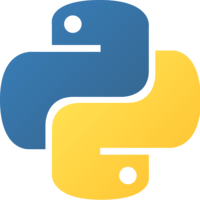This lesson is for members. Join us? Get access to all 3,000+ tutorials + a community with expert developers around the world.

Unlock This Lesson
1×
Become a member
to unlock all featuresLevel Up!

Access all courses & lessons on egghead today and lock-in your price for life.

Autoplay

Use K-nearest Neighbors To Find Similar Datapoints with Python and Scikit-learnScikit-Learnpython

We’ll continue with the iris dataset to implement k-nearest neighbors (KNN), which makes predictions about data based on similarity to other data instances. We'll visualize how the KNN algorithm works by making its predictions based on its neighbors' labels. We'll also examine the confusion matrix a bit further.

KNN can be used for both classification and regression problems.

KNN is good for low dimensional data (data without too many input variables). It is not good for unbalanced data sets, and it can be computationally expensive.CodeYou must be a Member to view code

Access all courses and lessons, track your progress, gain confidence and expertise.

Become a Member
and unlock code for this lessonDiscussTranscript

Instructor: From sklearn, we'll import datasets. We'll import our metrics. We'll import our train_test_split. From sklearn, we'll import neighbors. We'll also import matplotlib.pyplot as plt. We're working with the iris dataset, which is datasets.load_iris. We'll assign our X to be iris.data and our y to be iris.target.

Then we'll split our dataset up into training and test datasets. We'll say X_train, X_test, y_train, y_test equals train_test_split, pass it our X, and Y, and a test size -- which is the percentage of the data that we want to go into our test dataset -- we'll say 15, and our random state, let's say 2. Let's visualize how k-nearest neighbors works.

Let's plot by saying plt.scatter. Let's just plot the first two variables of X -- in Python, the syntax looks like that -- to take the first variable and the second variable. We could also pass in a color argument. The color will be based on the y labels. Then we have to type plt.show. Let's see how this looks. Here we can see our three classes of data.

The way k-nearest neighbors works is that it looks at a certain number of neighbors and predicts the label based on the labels of those neighbors. Let's see how this works in practice. Into our scatter plot, we'll add our test data, again only using the first two variables for simplicity's sake. We'll color these magenta. These are the data points we're making predictions for.

If we wanted to predict this magenta data point using the three nearest neighbors, we would see a yellow data point, a yellow data point, and a yellow data point. The k-nearest neighbors model would predict that this data point would be in the yellow class.

For this one here, if we used a k-nearest neighbors model with five neighbors, we would look at this turquoise one, this turquoise one, this yellow one, this turquoise one, and this turquoise one. The model would predict that this one is turquoise.

One other thing is that in order to prevent ties, it's a good idea to choose an even number of neighbors if you have an odd amount of classes and vice versa. It's also a good idea not to choose a number of neighbors that's a multiple of your amount of classes.

From here, we can say model equals neighbors.KNeighborsClassifier. We pass it the number of neighbors by saying n_neighbors equals, we'll say, 10. We can say model.fit and pass our X_train and y_train data. Then we can print the model's score by passing in the test data. Let's check that out. Not bad.

We can make our predictions by saying model.predict on our X_test data. We can also print our metrics.classification_report and passing in our accurate labels and our predicted labels. We can print our confusion matrix.

We can see the model looks pretty good. It predicted 11 out of 11 in class 0correctly. In class 1, it predicted five correctly, but it predicted one item to be in class 2. For class 2, it predicted all six correctly.